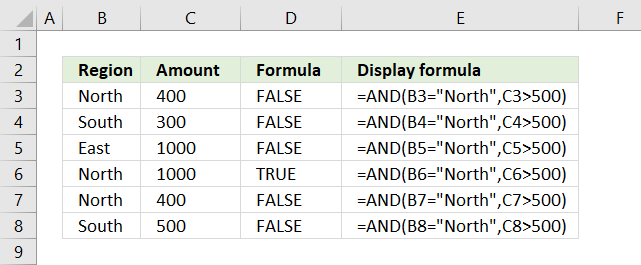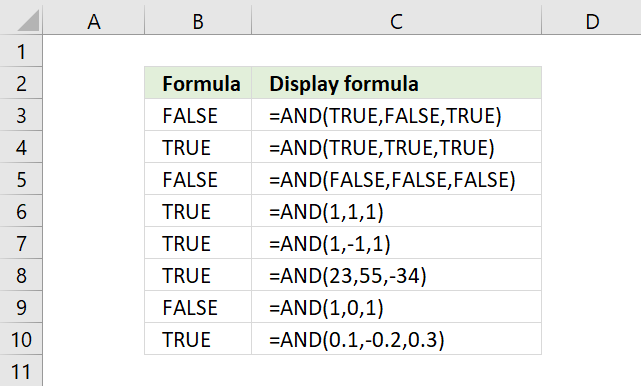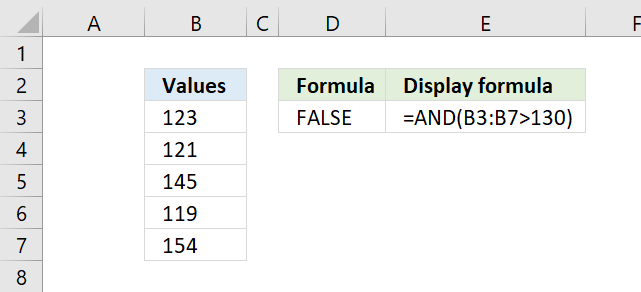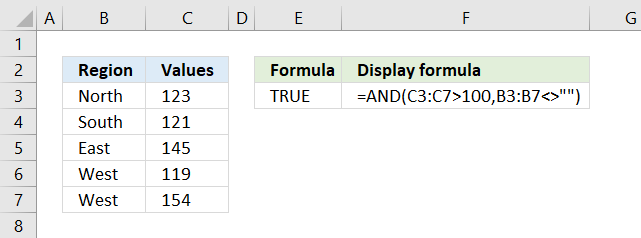Author: Oscar Cronquist Article last updated on March 27, 2018The image above demonstrates the AND function with two logical expressions. If the value in column B is equal to "North" and the value on the same row in column C is above 500 the AND function returns TRUE. These conditions are only met on row 6.

### Excel function syntax

AND(logical1, [logical2], ...)

The AND function allows you to perform a logical test in each argument and if all arguments return TRUE the AND function returns TRUE. If at least one argument returns FALSE the AND function returns FALSE.

### Arguments

logical1 - Required. A logical expression or a function/functions that returns a number.

[logical2] - Optional. Also a logical expression or a function/functions that returns a number. You can have up to 254 arguments.The AND function arguments can result in TRUE or FALSE, however, it also treats all numbers, both positive and negative, as TRUE.

The exception to that is 0 (zero) which is treated the same as FALSE.

### You can also enter the AND function as an array formula

The AND function also allows you to not only compare a single cell value but also an entire cell range.The formula in cell D3 checks if each value in cell range B3:B7 is larger than 130. Three values are not larger than 130 so the AND function returns FALSE.

AND(B3:B7>130)

becomes

AND({FALSE; FALSE; TRUE; FALSE; TRUE})

and returns FALSE.

To enter the formula above as an array formula, type the formula in a cell. Press and hold CTRL + SHIFT keys simultaneously, then press Enter once. Release all keys. The formula is now enclosed with curly brackets, they indicate you successfully entered the formula as an array formula. Don't enter the curly brackets yourself.The array formula above in cell E3 has two arguments containing a logical expression in each. The first argument checks if values in cell range C3:C7 are larger than 100.

C3:C7>100

becomes

{TRUE;TRUE;TRUE;TRUE;TRUE}

The second argument checks if values in cell range B3:B7 are not empty.

B3:B7<>""

becomes

{TRUE; TRUE; TRUE; TRUE; TRUE}

All values are TRUE so the AND function returns TRUE.

AND(C3:C7>100,B3:B7<>"")

becomes

AND({TRUE;TRUE;TRUE;TRUE;TRUE},{TRUE; TRUE; TRUE; TRUE; TRUE}) and returns TRUE.